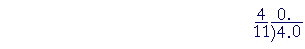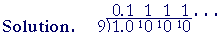S k i l l
i n
A R I T H M E T I C

Lesson 24  Section 2

# FRACTIONS INTO DECIMALS

## Exact versus inexact decimals

Back to Section 1

In the previous section we saw the most frequent
and therefore the most important decimal and percent equivalents. Nevertheless, we now ask the following:

 3. What is a general method for changing a fraction to a decimal? 4 11 Divide the numerator by the denominator.

Example 1.   Writeas a decimal.

Solution.= 4 ÷ 11.  As they said in the Little Red School House:

"Let 4 fall into the house"11 does not go into 4.  Write 0 in the quotient, place a decimal point,and add a 0 onto the dividend. (Lesson 12)

"11 goes into 40 three (3) times (33) with 7 left over."11 goes into 70 six (6) times (66) with 4 left over."Since we are dividing 11 into 40 again, we see that this division will never be exact.  We will have 36 repeated as a pattern:

 4 11 = 0.363636. . .

By writing three dots (called ellipsis), we mean, "No decimal forwill ever be complete or exact. However we can approximate it with as many decimal digits as we please according to the indicated pattern; and the more decimal digits we write, the closer we will be to."

That is a fact. It is possible to produce that decimal approximation and thus know it and utilize it. We have not said that .363636 goes on forever, because we cannot produce an infinite sequence of digits nor can we be aware of one. It is nothing but a thought.

We are taught of course that .363636 goes on forever, and so we think that's mathematics—that's the way things are. We do not realize that it is a man-made conceit.

This writer asserts that what we can actually bring into this world—.363636—has more being for mathematics than what is only an idea. We can logically produce a decimal approximation.

What is more, infinite decimals are not required to solve any problem in arithmetic or calculus; they have no consequences and therefore they are not even necessary.

Even if we imagine that the decimal did go on forever, then 1) it would never end and would never equal; and 2) it would not be a number. Why not? Because, like any number, a decimal has a name. It is not that we will never finish naming an infinite sequence of digits. We cannot even begin.

And so we cannot expressexactly as a decimal. Therefore if we want to use that number as a decimal, we must approximate it.  Let us approximate it with three decimal digits (Lesson 12):

 4 110.364
 Example 2.   Write 5 4 11 as a decimal.

Answer.  According to what we just saw:

 5 4 115.364
 Example 3.   Write as a decimal: 309 16 .

Solution.  We are to divide 309 by 16.

 Now, 320 16 = 20.  Therefore,
 309 16 = 320 − 11     16
 = 20 − 1116 (Lesson 11)
 = 19 5 16 (Lesson 21)
 = 19.3125 (Section 1)

Exact versus inexact decimals

Any decimal forwill be inexact. But the decimal for ¼, which is .25, is exact.

 The decimal  .363636  in and of itself is exact. But as a value for 4 11 , it is inexact.

Fractions, then, when expressed as decimals, will be either exact or inexact.  Inexact decimals nevertheless exhibit a pattern of digits.  The pattern foris .3636363636.

Which fractions—in lowest terms—will have exact decimals?  Only those whose denominators could be multiplied to become a power of 10. For they are the denominators that a decimal fraction is understood to have. They are the numbers whose only factors are 2's and/or 5's; which are the only factors of the powers of 10.

Here are a few of the numbers that are composed only of 2's or 5's:
2, 4, 5, 8, 10, 16, 20, 25, 40, 50, 250, 400. A fraction with any of those denominators will have an exact decimal.

A fraction in lowest terms with denominator 6 will not have an exact decimal, because 6 = 2 × 3.  It is not possible to multiply 2 × 3 so that it becomes a power of 10.

Example 4.

 a)   Show the decimal pattern that 19 generates.9 goes into 1 zero (0) times.

9 goes into 10 one (1) time with 1 left over.

Again, 9 goes into 10 one (1) time with 1 left over.

And so on. This division will never be exact—we will keep getting 1's in the quotient.

 19 = 0.111111. . .
 b)   Use that value for 19 to find the value of 89 .
 Solution. 89 = 8 × 19 =  8 × 0.111111. . . =  0.8888888. . .
 c)   Round off 89 to three decimal digits.
 890.889.

See Problem 15 at the end the Lesson.

 Example 5.  Calculator problem.   Write as a decimal: 7396 .

Answer.  Divide 73 by 96.  Press

 73 ÷ 96 =

Displayed is

 0.760417

Therefore, to three decimal digits,

 73960.76

Example 6.   In a class of 52 students, 29 were women.

a)  What fraction were women?

Answer.  Since 29 out of  52 were women, thenwere women.

b)  Use a calculator to express that fraction as a decimal.

 2 9 ÷ 5 2 =

See

 0.557692

This is approximately  .558.

c)  What percent were women?

Answer.   To change a number to a percent, multiply it by 100.

.558 = 55.8%

In summary, look at what we have done:

 29 out of  52 = 2952 = 29 ÷ 520.558 = 55.8%.

"Out of," with a calculator, always signifies division; division of a smaller number by a larger.

Please "turn" the page and do some Problems.

or

Continue on to the next Lesson.

Section 1 of this Lesson